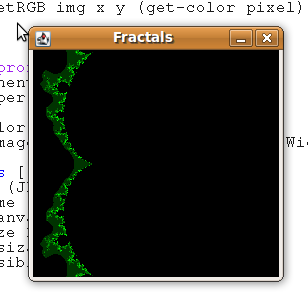## Sunday, 1 February 2009

### Mandlebrot Fractals

The Mandlebrot Set is probably the most famous set of fractals. The maths behind it is dead simple and with just a few lines of code you can get some impressive results.

For any given pixel, you can work out a colour value thus:

(def *max-iteration* 512)

(defn process-pixel [x y]
((fn [x y xc yc accum]
(let [x1 (+ (- (* x x) (* y y)) xc)
y1 (+ (* 2 x y) yc)
sq (+ (* x1 x1) (* y1 y1))]
(cond
(> accum *max-iteration*) *max-iteration*
(> sq 2.0) accum
:else (recur x1 y1 xc yc (inc accum))))) x y x y 0))

The harder part is translating a number between 0 and `*max-iteration*` into a decent range of colours. I'll ignore this for now and stick with green!Source code for version 0.1 is here. Next on the list:

• Make it look like the Math - write complex number library
• Make it run fast - optimize (uses more cores, minimize type coercions)
• Make it look nice - find a better colour mapping function